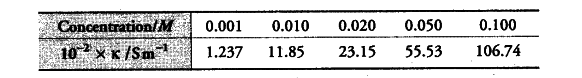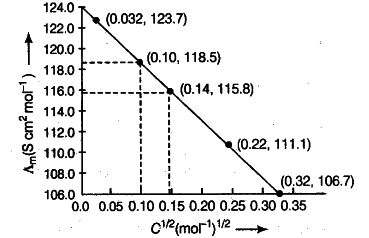# The conductivity of NaCl

The conductivity of NaCl at 298 K has been determined at different concentrations and the results are given belowCalculate A_{ m } for all concentrations and draw a plot between A_{ m } and {{C}^{1/2}}

According to the question, the given values are 1 S {{cm}^{-1}} = 100 S {{m}^{-1}}

So, the graph between A_{ m } and {{C}^{1/2}} is shown along side.